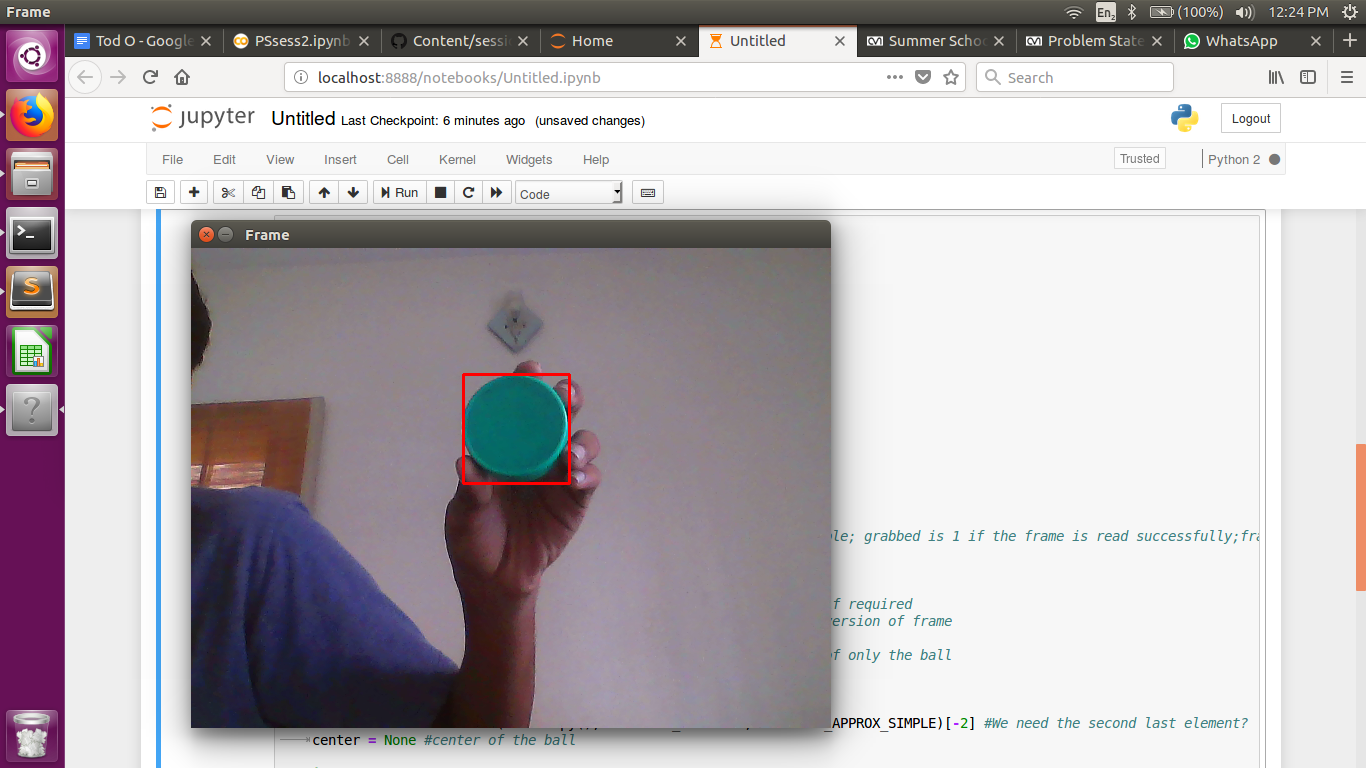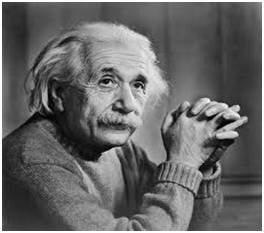# Problem Statements on basic OpenCV

Now that we’re done with the first session and the basics of Computer Vision and OpenCV, we have some problem statements for you to delve into. Do go through our session notebook if you missed it or don’t remember what was covered.

We are going to use OpenCV to draw bounding boxes around a ball of a specific colour. The output would finally run in real time and would look like:So let’s get started!!

• First learn to use cv2.VideoCapture() to access images from your webcam. So you should be able to store each frame in a variable called ‘frame’

The code will look a bit like:

Import cv2

# Create VideoCapture object here
cap = ......

while(True):
# Read image from webcam here
ret, frame = ………

# frame now stores the image

# Show output using cv2.imshow()
.........

# Exit loop if ‘q’ is pressed
if cv2.waitKey(1) & 0xFF == ord('q'):
break

# Release the VideoCapture object and destroy all windows
cap.release()
cv2.destroyAllWindows()

• We are going detect only green objects. This is done easily in HSV space. In the loop first convert the image from BGR to HSV space using cv2.cvtColor().

The lower and upper ranges of green in HSV space are (29, 86, 6) and (100, 255, 255) respectively. Use cv2.inRange() to create a mask such that only those regions of the images which were green will be seen in the mask. Use cv2.imshow() on mask to see the output

• Do erosion followed by dilation on the mask to remove noise.

• We are now going to find contours in our mask. Find out how the cv2.findContours() function works and use it to do so. It will look a bit like:

cnts = cv2.findContours(mask.copy(), cv2.RETR_EXTERNAL,cv2.CHAIN_APPROX_SIMPLE)[-2]
# 'mask' is the output of erosion followed by dilation.
# 'cnts' is now a list of contours

• Use the max() function to choose the largest contour based on area. max() accepts two arguments: the first one is the list of contours and the second one (key) is the criteria for choosing the maximum. (Here it will be cv2.contourArea)
C_max = max(cnts,key = cv2.contourArea)
# 'cnts' was the list of contours
# 'C_max' is stores the largest contour

• Use cv2.boundingRect() to get the coordinates of one point on the rectangle bounding the contour and its height and width. Now use the cv2.rectangle() function to draw the rectangle on the frame. Use cv2.imshow() and see the output.

## Task 2: Edge Detection using Difference of Gaussians

In our last session you would have come across the Gaussian Filter for blurring an image. If you use two Gaussians filters of different sizes (having different standard deviations) and find their difference, it acts like a band pass filter and can detect edges.

So try this out on the following image:Perform Gaussian Blurring with kernels of size 5x5 and 9x9 and find their difference and see the output.

Check out this colab link for this Problem Statement

Make a copy of it in your drive and then start working on it

## Task 4: Template Matching using Histograms

The task is to recognise the orange and white barrels in this video. You will be using histogram backprojection to do so. Try to understand how cv2.calcBackProject() works for this.

The following are the steps you will roughly have to follow:

• Get an image of the region of interest(the barrel), convert this to HSV from RGB. This is what you will be using for template matching.

• Open your video using cv2.VideoCapture(), and convert your frame to HSV

• Calculate the histogram of your object using the cv2.calcHist() function. Normalize your histogram, and apply histogram backprojection using cv2.normalize() and cv2.calcBackProject().

• Let the result after calculating backproject be ‘res’. Now to visualize ‘res’ better, we shall convolve with a circular disc.

disc = cv2.getStructuringElement(cv2.MORPH_ELLIPSE, (5,5))
cv2.filter2D(res, -1, disc, res) #res is the matrix obtained after back projection

• Threshold your image. Try out different values for best results.

• Merge the thresholded matrices to get a 3 channel image

final = cv2.merge((thresh,thresh,thresh))

• Perform a bitwise or of ‘final’ with the target image and display the output
result = cv2.bitwise_or(target_img, final)


If this gives good results you can also go ahead and try to identify the white lines bounding the path.

Use the comment section to ask doubts or raise any issues you face. Kindly refrain from putting up solutions though :P

Categories:

Updated: